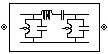# LC Bandpass Pi

Model LC bandpass pi network

• Library:
• RF Blockset / Equivalent Baseband / Ladder Filters

•## Description

The LC Bandpass Pi block models the LC bandpass pi network described in the block dialog box, in terms of its frequency-dependent S-parameters.

For each inductor and capacitor pair in the network, the block first calculates the ABCD-parameters at each frequency contained in the vector of modeling frequencies. For each series pair, A = 1, B = Z, C = 0, and D = 1, where Z is the impedance of the series pair. For each shunt pair, A = 1, B = 0, C = Y, and D = 1, where Y is the admittance of the shunt pair.

The LC Bandpass Pi block then cascades the ABCD-parameters for each series and shunt pair at each of the modeling frequencies, and converts the cascaded parameters to S-parameters using the RF Toolbox™ `abcd2s` function.

See the Output Port block for information about determining the modeling frequencies.

The LC bandpass pi network object is a two-port network as shown in the following circuit diagram.[L1, L2, L3, L4, ...] is the value of the `'L'` property, and [C1, C2, C3, C4, ...] is the value of the `'C'` property.

## Parameters

expand all

### Main

Vector containing the inductances, in order from source to load, of all inductors in the network. The inductance vector must contain at least three elements. All values must be strictly positive.

Vector containing the capacitances, in order from source to load, of all capacitors in the network. Its length must be equal to the length of the vector you provide in the Inductance parameter. All values must be strictly positive.

### Visualization

When Source of frequency data is `User-specified`, specify as a vector of frequencies in the Frequency data parameter.

Frequency data range, specified as a vector in hertz.

#### Dependencies

To set this parameter, first select `User-specified` in Source of amplifier gain. This selection activates the Visualization Tab which contains Source of frequency data

Reference impedance of the coaxial transmission line, specified as a scalar in ohms.

Type of data plot that you want to produce with your data specified as :

• `X-Y plane` — Generate a Cartesian plot of your data versus frequency. To create linear, semi-log, or log-log plots, set the Y scale and X scale accordingly.

• `Composite data`—The composite data plot automatically generates four separate plots in one figure window, showing the frequency dependence of several parameters.

• `Polar plane` — Generate a polar plot of your data. The block plots only the range of data corresponding to the specified frequencies.

• `Z Smith chart`, ```Y Smith chart```, and ```ZY Smith chart``` — Generate a Smith® chart of your data. The block plots only the range of data corresponding to the specified frequencies.

Type of parameters to plot, specified as one of the following `S11`, `S12`, `S21`, `S22`, `GroupDelay`, `GammaIn`, `GammaOut`, `VSWRIn`, `VSWROut`, `OIP3`, `IIP3`, `TF1`, `TF2`, `TF3`, `Gt`, `Ga`, `Gp`, `Gmag`, `Gmsg`, `GammaMS`, `GammaML`, `K`, `Delta`, `Mu` or `MuPrime`. When noise is spectral `NF`, `NFactor` and `NTemp` plotting is possible.

Type of parameters to plot, specified as one of the following `S11`, `S12`, `S21`, `S22`, `GroupDelay`, `GammaIn`, `GammaOut`, `VSWRIn`, `VSWROut`, `OIP3`, `IIP3`, `TF1`, `TF2`, `TF3`, `Gt`, `Ga`, `Gp`, `Gmag`, `Gmsg`, `GammaMS`, `GammaML`, `K`, `Delta`, `Mu` or `MuPrime`. When noise is spectral `NF`, `NFactor` and `NTemp` plotting is possible.

Plot format, specified as one of the following ```Magnitude (decibels)```, `Angle (degrees)`, `Real`, or `Imaginary`.

Plot format, specified as one of the following ```Magnitude (decibels)```, `Angle(degrees)`, `Real`, or `Imaginary`.

Parameter, specified as `Freq`. This parameter determines the data for x-axes on the X-Y plane plot.

Plot format, specified as one of the following `Hz`, `Auto`, `KHz`, `MHz`, `GHz` or `THz`.

Y-axis scale, specified as `Linear` or `Log`.

X-axis scale, specified as `Linear` or `Log`.

Plot the specified data using the plot button.

 Ludwig, Reinhold and Pavel Bretchko. RF Circuit Design: Theory and Applications. Prentice-Hall, 2000.

 Zverev, Anatol I.Handbook of Filter Synthesis . John Wiley & Sons, 1967.